2020/08/24 16:13

F1 值

sklearn 的评估函数

pyspark 的评估函数

tensorflow 的评估函数

# 基本概念

TP（True Positives)：真正例，预测为正例而且实际上也是负例；

FP（False Positives)：假正例，预测为正例然而实际上却是负例；

FN（false Negatives)：假负例，预测为负例然而实际上却是正例；

TN（True Negatives)：真负例，预测为负例而且实际上也是负例。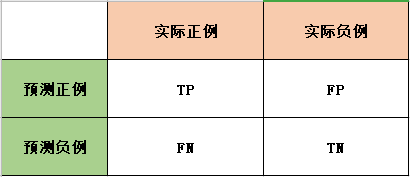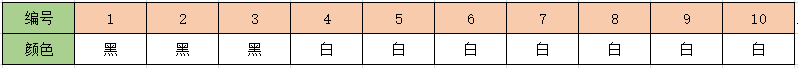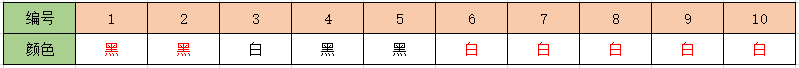TP：2个， 预测是黑色的，实际就是黑色的，即黑字红色部分。

FP：2个，预测是黑色的，实际是白色的，即黑字黑色部分，即编号4和5。

FN：1个，预测是白色的，实际是黑色的，即白字黑色部分，即编号3。

TN：5个。预测是白色的，实际是白色的，即白字红色部分。

# F1 值

1、什么情况下精确率很高但是召回率很低？

2、什么情况下召回率很高但是精确率很低？

F1值就是中和了精确率和召回率的指标：

# sklearn 的评估函数

from sklearn.metrics import accuracy_score, precision_score, recall_score, f1_score

y_true = [1, 1, 1, 0, 0, 0, 0, 0, 0, 0]
y_pred = [1, 1, 0, 1, 1, 0, 0, 0, 0, 0]
print("acc:", accuracy_score(y_true, y_pred))
print("p:", precision_score(y_true, y_pred))
print("r:", recall_score(y_true, y_pred))
print("f1:", f1_score(y_true, y_pred))

acc: 0.7
p: 0.5
r: 0.6666666666666666
f1: 0.5714285714285715

# pyspark 的评估函数

from pyspark.mllib.evaluation import MulticlassMetrics
from pyspark import SparkConf, SparkContext

conf = SparkConf() \
.setMaster("local") \
.setAppName("Metrics-test")
sc = SparkContext(conf=conf)

predictionAndLabels = sc.parallelize([  #(预测值，真实值)
(1.0, 1.0),
(1.0, 1.0),
(0.0, 1.0),
(1.0, 0.0),
(1.0, 0.0),
(0.0, 0.0),
(0.0, 0.0),
(0.0, 0.0),
(0.0, 0.0),
(0.0, 0.0)])

metrics = MulticlassMetrics(predictionAndLabels)

print("acc:", metrics.accuracy)
print("p:", metrics.precision(1.0)) # 必须传入label值，否则统计的是类别0和类别1的均值
print("r:", metrics.recall(1.0))
print("f1", metrics.fMeasure(1.0))


# tensorflow 的评估函数

import tensorflow as tf
train_graph = tf.Graph()
with train_graph.as_default():
labels = tf.constant([1, 1, 1, 0, 0, 0, 0, 0, 0, 0])
predicts = tf.constant([1, 1, 0, 1, 1, 0, 0, 0, 0, 0])

# 返回的是一个二元组tuple
accuracy = tf.metrics.accuracy(labels, predicts)
precision = tf.metrics.precision(labels, predicts)
recall = tf.metrics.recall(labels, predicts)
f1 = tf.metrics.mean((2 * precision * recall) / (precision + recall), name='f1_score')

with tf.Session(graph=train_graph) as sess:
sess.run(tf.local_variables_initializer())
result = sess.run([accuracy, precision, recall, f1])
print(result)

[(0.0, 0.7), (1.0, 0.5), (1.0, 0.6666667), (0.0, 0.57142854)]

# 多分类下的评估

from sklearn.metrics import accuracy_score, precision_score, recall_score, f1_score

y_true = [1, 1, 1, 0, 0, 0, 0, 0, 0, 0]
y_pred = [1, 1, 0, 1, 1, 0, 0, 0, 0, 0]
print("p:", precision_score(y_true, y_pred, average='macro'))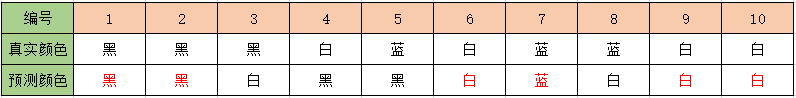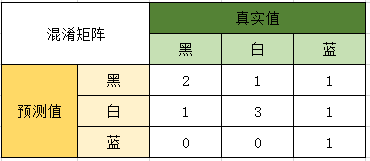P黑=2/4

P白=3/5

P蓝=1/1

from sklearn.metrics import accuracy_score, precision_score, recall_score, f1_score

y_true = [1, 1, 1, 0, 2, 0, 2, 2, 0, 0]
y_pred = [1, 1, 0, 1, 1, 0, 2, 0, 0, 0]
print("p:", precision_score(y_true, y_pred, average='macro'))
print("acc:", accuracy_score(y_true, y_pred))

p: 0.7000000000000001
acc: 0.6### 作者的其它热门文章

0
0 收藏

0 评论
0 收藏
0